# Adding fractions with tape diagrams and area model

This is the third of a multi-part series of posts sharing ideas for how to teach fraction concepts and operations through the use of visual representations. There is tons of evidence that students learn math better when the math is accompanied by visuals, so let’s dig into fractions…

Suppose we want to add $$\frac{2}{5}$$ and $$\frac{2}{3}$$ and represent it with a tape diagram.

Modeling both  $$\frac{2}{5}$$  and  $$\frac{2}{3}$$ with tapes, we see that $$\frac{2}{5} +\frac{2}{3}$$  cannot be added together because they do not yet have equal sized pieces.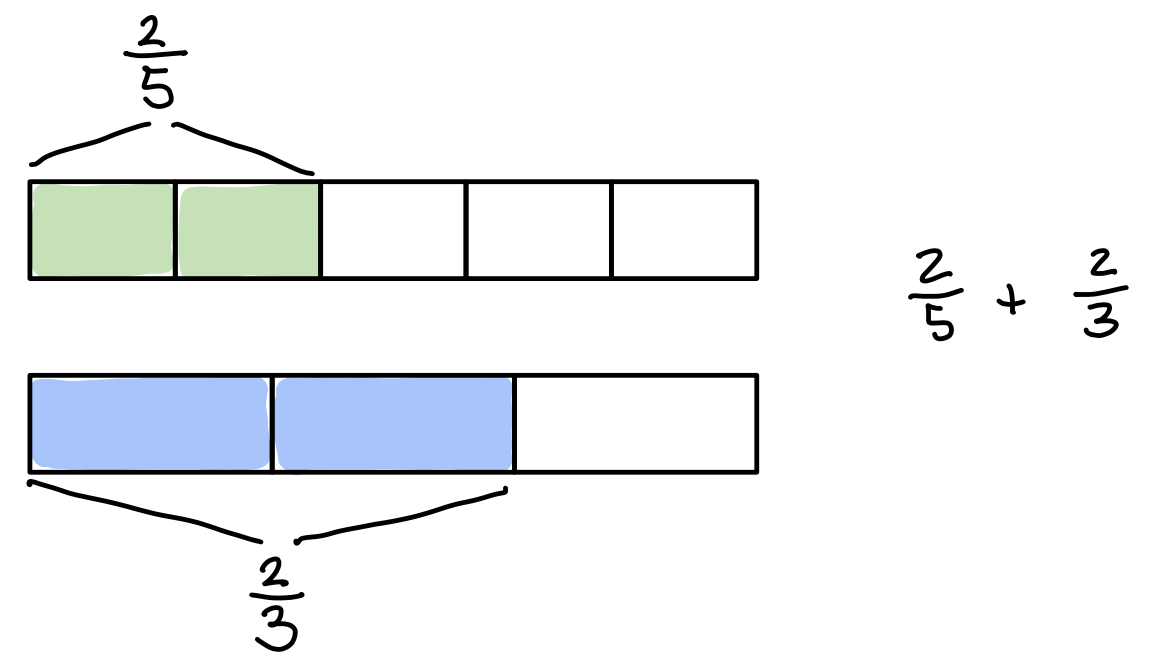We can cut the pieces into identical sizes by cutting each fifth into 3 pieces and each third into 5 pieces. This results in each tape being cut into 15 pieces…our common denominator!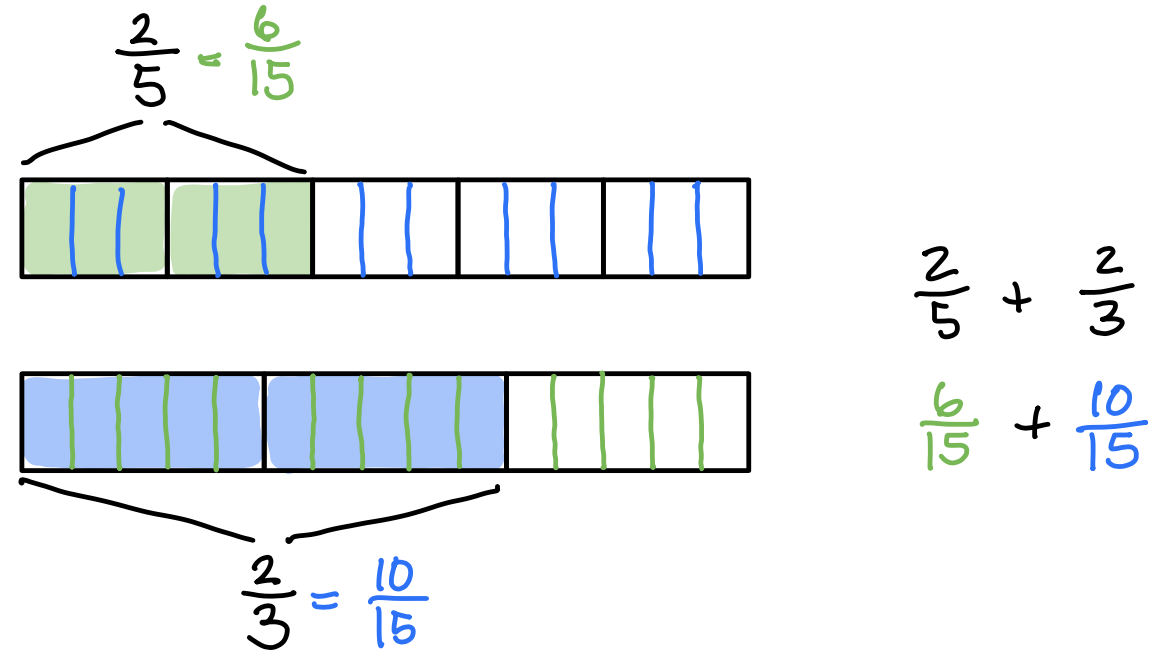Once the equal sizes are created, $$\frac{2}{5} +\frac{2}{3}$$ now becomes

$$\frac{6}{15} +\frac{10}{15}$$, which equals $$\frac{16}{15}$$.

Since $$\frac{16}{15}$$ is an improper fraction, it can be rewritten as the mixed number $$1\frac{1}{15}$$.

We can see that $$1\frac{1}{15}$$ makes sense because we can move pieces from one tape to to the other tape to create one full tape (1 whole).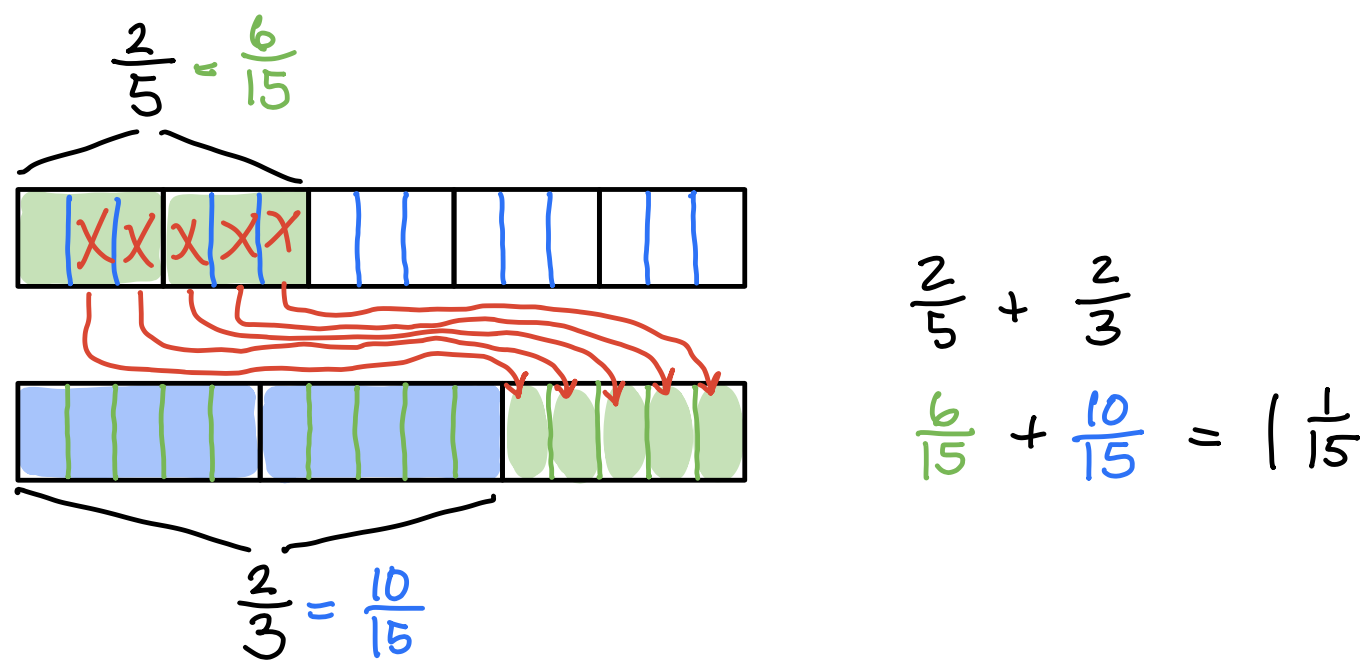Numerically, we would write the entire process like this…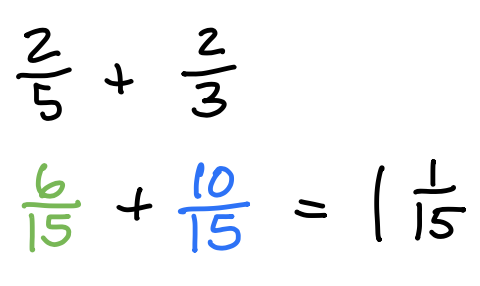What about using the area model? Let’s solve $$\frac{2}{3} + \frac{3}{4}$$.

First, let’s model the two fractions. But…you will notice that we made vertical slices to model $$\frac{2}{3}$$ and we used horizontal slices to model $$\frac{3}{4}$$. This is intentional!Then we use horizontal lines to cut each of the thirds into four pieces. We use vertical lines to cut each of the fourths into three pieces. Now both rectangles have been cut into 12ths. Also, we see that $$\frac{2}{3} + \frac{3}{4}$$ has been renamed as $$\frac{8}{12} + \frac{9}{12}$$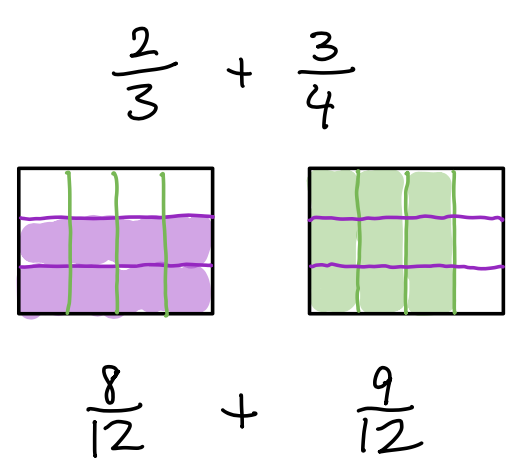The final answer is $$1\frac{5}{12}$$, which makes sense since we can move some of the green pieces to the left to create 1 whole and 5 green pieces left over.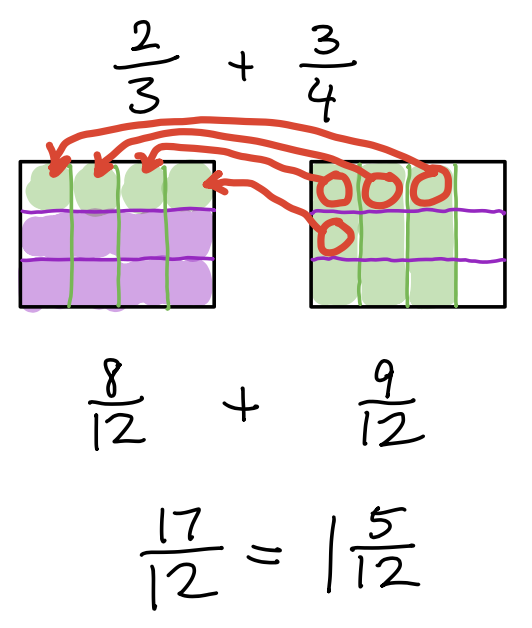Here is a video using tape diagrams for addition:

Here is a video using area model for addition:

1.Marie David says: